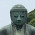## 2011/01/18

### Valuation of CDO with equal amount

Bank of Japan(BOJ) publish research paper regularly.
And, they issued very interesting paper about valuation of CDO recently.

They introduced copula for pricing of CDO,and discussed how different CDO spreads were with using different copula for pricing.
I would like to reproduce their result (especially,P23-Table7)

The condition of calculation is following that
• number of debt（NUM.REFDEBT）:=100
• maturity(MATURITY):=5 year
• recovery rate(RECOVERY.RATE):=40%（constant value）
• probability of default (DEFAULT.PROBABILITY):=5％（in 5 years）
• parameter of nomal copula ρ:=0.15
• parameter of clayton copula α:=0.21
They apporoximated their valuation formula for easy calculation(equation (27))
(They assumed that CDO spread were paid as discounted bond at the begging.)

I simulated valuation of CDO with their method.
The result is following that
copula/trancheEquitymezzanineseniorsuper senior
normal1,145.4262.490.520.000
t(20)1,055.2886.072.180.004
t(6)896.74126.448.560.044
t(3)733.31165.9023.560.191
clayton857.64135.7312.830.084
This table reproduce their result(P23-Table7).
And, In senior or super senior,you can understand that the CDO spread which is evaluated by normal copula is lower than the others. It means that normal copula is inadequate in financial crisis.
I show you my programming code（by R language).
If you copy and run my source code, you can duplicate my result easily.
Before you run, please install "copula"package.

```library(copula)
#function for CDO spread calculation
SpreadOfCDO <- function(copula, default.probability, maturity,
recovery.rate, attachment, detachment, num.path, num.refdebt)
{
random.copula <- rcopula(copula,num.path)
num.default <- rowSums(random.copula < default.probability)
loss.refdebt <- (1-recovery.rate)/num.refdebt*num.default
loss.tranche <- (pmax(loss.refdebt - attachment,0)-pmax(loss.refdebt - detachment,0))/(detachment-attachment)
expectation.loss.tranche <- sum(loss.tranche)/num.path
spread <- -1/maturity*log(1-expectation.loss.tranche)
return(spread)
}
################  main  ##################
#parameter
NUM.PATH <- 10^3
NUM.REFDEBT <- 100
DEFAULT.PROBABILITY <- 0.05
MATURITY <- 5
RECOVERY.RATE <- 0.4
#copulas which I want to compare.
COPULA <- list(normalCopula(0.15, dim = NUM.REFDEBT),
tCopula(0.15, dim = NUM.REFDEBT, df = 20),
tCopula(0.15, dim = NUM.REFDEBT, df = 6),
tCopula(0.15, dim = NUM.REFDEBT, df = 3),
claytonCopula(0.21, dim = NUM.REFDEBT)
)
#define for easy programming
SpreadOfCDOWithFixedParameter <- function(copula,attachment, detachment){
SpreadOfCDO(copula, DEFAULT.PROBABILITY, MATURITY,
RECOVERY.RATE, attachment, detachment, NUM.PATH, NUM.REFDEBT)
}
result <- list()
#tranche:equity
result[] <- sapply(COPULA,SpreadOfCDOWithFixedParameter,0.0,0.06)
#tranche:mezzanine
result[] <- sapply(COPULA,SpreadOfCDOWithFixedParameter,0.06,0.18)
#tranche:senior
result[] <- sapply(COPULA,SpreadOfCDOWithFixedParameter,0.18,0.36)
#tranche:super senior
result[] <- sapply(COPULA,SpreadOfCDOWithFixedParameter,0.36,1)
#convert to matrix, and chenge unit to "bp"
result <- 10^4*do.call("cbind", result)
colnames(result) <- c("equity","mezzanine","senior","super senior")
rownames(result) <- c("normal","t(20)","t(6)","t(3)","clayton")
result```
Created by Pretty R at inside-R.org

#### 4 件のコメント:

1.Hi, this programme is great - however I was wondering if you had an alternative that had a fixed CDO spread and a variable upfront payment, as is often used with Equity tranches. Also, can this be adapted to Base Correlation?

2.Thank you for your comment.
But, I'm sorry that I do not have that alternative one.

3.ok no problem - what does the num.path mean in your equation? And why did you choose 10^3. Also, can you incorporate an integration method such as gauss-Hermite quadrature into this?

4.the num.path means just "the number of monte-carlo path" to calculate CDO spread.
As you may know, R language does not have a high speed to calculate.This is why I choose 10^3 as this value.